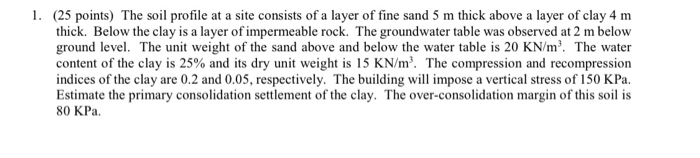# 1. (25 points) The soil profile at a site consists of a layer of fine sand...

###### Question:1. (25 points) The soil profile at a site consists of a layer of fine sand 5 m thick above a layer of clay 4 m thick. Below the clay is a layer of impermeable rock. The groundwater table was observed at 2 m below ground level. The unit weight of the sand above and below the water table is 20 KN/m'. The water content of the clay is 25% and its dry unit weight is 15 KN/m'. The compression and recompression indices of the clay are 0.2 and 0.05, respectively. The building will impose a vertical stress of 150 kPa. Estimate the primary consolidation settlement of the clay. The over-consolidation margin of this soil is 80 KPa.

#### Similar Solved Questions

##### Problem 1: Oscillation Problem Part 1: A spring that you find in the lab is characterized...
Problem 1: Oscillation Problem Part 1: A spring that you find in the lab is characterized by the graph below. A. What is the unstretched length for the spring? B. What is the spring constant for this springi C. Write the equation that describes the spring force Fup in terms of the spring length L sp...
Chapter 12 Quiz 0 Seved Help Save & Exit Submit TB MC Qu. 12-59 Lusk Corporation produces and sells ... Lusk Corporation produces and sells 15,300 units of Product X each month. The selling price of Product X is $23 per unit, and variable expenses are$17 per unit. A study has been made concerni...